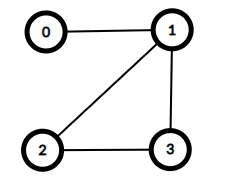시간 제한 메모리 제한 제출 정답 맞은 사람 정답 비율
1 초 1024 MB 64 28 25 45.455%

## 문제

Gardens by the Bay is a large nature park in Singapore. In the park there are $n$ towers, known as supertrees. These towers are labelled $0$ to $n-1$. We would like to construct a set of zero or more bridges. Each bridge connects a pair of distinct towers and may be traversed in either direction. No two bridges should connect the same pair of towers.

A path from tower $x$ to tower $y$ is a sequence of one or more towers such that:

• the first element of the sequence is $x$,
• the last element of the sequence is $y$,
• all elements of the sequence are distinct, and
• each two consecutive elements (towers) in the sequence are connected by a bridge.

Note that by definition there is exactly one path from a tower to itself and the number of different paths from tower $i$ to tower $j$ is the same as the number of different paths from tower $j$ to tower $i$.

The lead architect in charge of the design wishes for the bridges to be built such that for all $0 \leq i, j \leq n-1$ there are exactly $p[i][j]$ different paths from tower $i$ to tower $j$, where $0 \leq p[i][j] \leq 3$.

Construct a set of bridges that satisfy the architect's requirements, or determine that it is impossible.

## 구현

You should implement the following procedure:

int construct(int[][] p)

• $p$: an $n \times n$ array representing the architect's requirements.
• If a construction is possible, this procedure should make exactly one call to build (see below) to report the construction, following which it should return $1$.
• Otherwise, the procedure should return $0$ without making any calls to build.
• This procedure is called exactly once.

The procedure build is defined as follows:

void build(int[][] b)

• $b$: an $n \times n$ array, with $b[i][j]=1$ if there is a bridge connecting tower $i$ and tower $j$, or $b[i][j]=0$ otherwise.
• Note that the array must satisfy $b[i][j]=b[j][i]$ for all $0 \leq i,j \leq n-1$ and $b[i][i] = 0$ for all $0 \leq i \leq n-1$.

## 제한

• $1 \leq n \leq 1000$
• $p[i][i] = 1$ (for all $0 \leq i \leq n-1$)
• $p[i][j] = p[j][i]$ (for all $0 \leq i, j \leq n-1$)
• $0 \leq p[i][j] \leq 3$ (for all $0 \leq i, j \leq n-1$)

## 예시 1

Consider the following call:

construct([[1, 1, 2, 2], [1, 1, 2, 2], [2, 2, 1, 2], [2, 2, 2, 1]])


This means that there should be exactly one path from tower $0$ to tower $1$. For all other pairs of towers $(x, y)$, such that $0 \leq x < y \leq 3$, there should be exactly two paths from tower $x$ to tower $y$.

This can be achieved with $4$ bridges, connecting pairs of towers $(0, 1)$, $(1, 2)$, $(1, 3)$ and $(2, 3)$.

To report this solution, the construct procedure should make the following call:

• build([[0, 1, 0, 0], [1, 0, 1, 1], [0, 1, 0, 1], [0, 1, 1, 0]])It should then return $1$.

In this case, there are multiple constructions that fit the requirements, all of which would be considered correct.

## 예시 2

Consider the following call:

construct([[1, 0], [0, 1]])


This means that there should be no way to travel between the two towers. This can only be satisfied by having no bridges.

Therefore, the construct procedure should make the following call:

• build([[0, 0], [0, 0]])

After which, the construct procedure should return $1$.

## 예시 3

Consider the following call:

construct([[1, 3], [3, 1]])


This means that there should be exactly $3$ paths from tower $0$ to tower $1$. This set of requirements cannot be satisfied. As such, the construct procedure should return $0$ without making any call to build.

## 서브태스크

번호 제한
1 11

$p[i][j] = 1$ (for all $0 \leq i, j \leq n-1$)

2 10

$p[i][j] = 0$ or $1$ (for all $0 \leq i, j \leq n-1$)

3 19

$p[i][j] = 0$ or $2$ (for all $i\neq j$, $0 \leq i, j \leq n-1$)

4 35

$0 \leq p[i][j] \leq 2$ (for all $0 \leq i, j \leq n-1$) and there is at least one construction satisfying the requirements.

5 21

$0 \leq p[i][j] \leq 2$ (for all $0 \leq i, j \leq n-1$)

6 4